# Algebra II : Quadratic Formula

## Example Questions

1 2 3 4 6 Next →

### Example Question #1641 : Algebra Ii

Determine a root: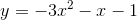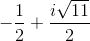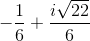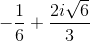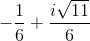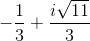Explanation:

Identify the coefficients given the equation in standard form: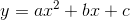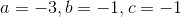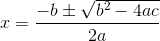Substitute the coefficients.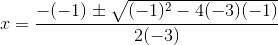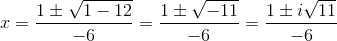These two roots are complex and either is a valid answer.

The answer is:### Example Question #1642 : Algebra Ii

Determine a possible root for: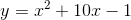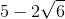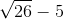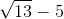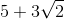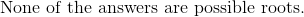Explanation:Identify and substitute the terms.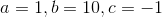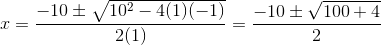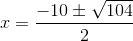Factor the radical using factors of perfect squares.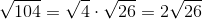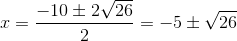These two answers are possible roots.

The answer is:### Example Question #193 : Solving Quadratic Equations

Find the roots of the quadratic function,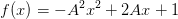Whereis any real number constant not equal to zero.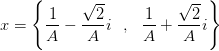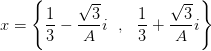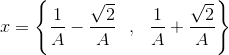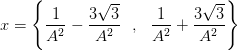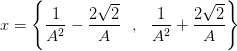Explanation:To find the roots set the function to zero: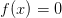,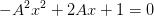(1)

_________________________________________________________________

Reminder

Recall that for a quadratic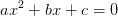the general formula for the solution in terms of the constant coefficients is given by: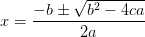(2)

_____________________________________________________________

Use equation (2) to write a solution for equation (1).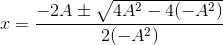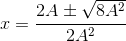If we simplify the right-hand term in the numerator we obtain: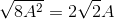So now we have for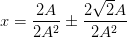After all the cancellations in the expression above we obtain: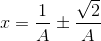Therefore, the solution set for this equation is:### Example Question #194 : Solving Quadratic Equations

Find the roots of the quadratic function,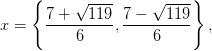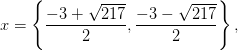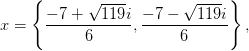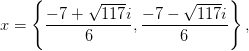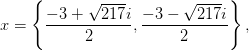Explanation: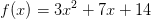The roots are the values offor which: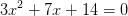______________________________________________________________

Reminder

Recall that for a quadraticthe general formula for the solution in terms of the constant coefficients is given by:_____________________________________________________________

Use the quadratic formula to find the roots.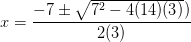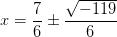Notice that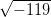is not a real number, and therefore the roots will be complex numbers.

Using the definition of the imaginary unit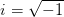we can rewriteas follows,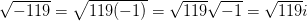Now we can write the solutions to this problem in the form: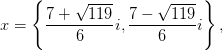### Example Question #193 : Solving Quadratic Equations

Find the roots using the quadratic formula.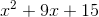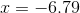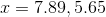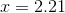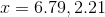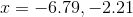Explanation: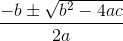For this problem

a=1, coefficient of x^2 term

b=9, the coefficient of the x term

c=15, the constant term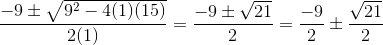solving the expression shows the roots of -6.79 and -2.21

1 2 3 4 6 Next →

### All Algebra II Resources